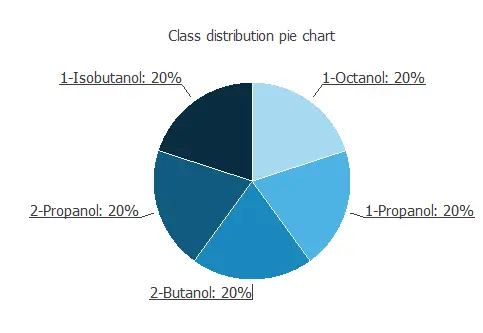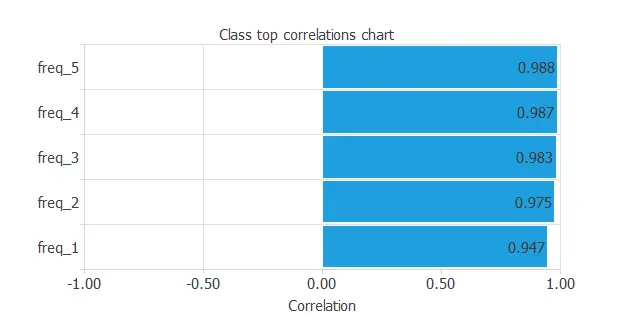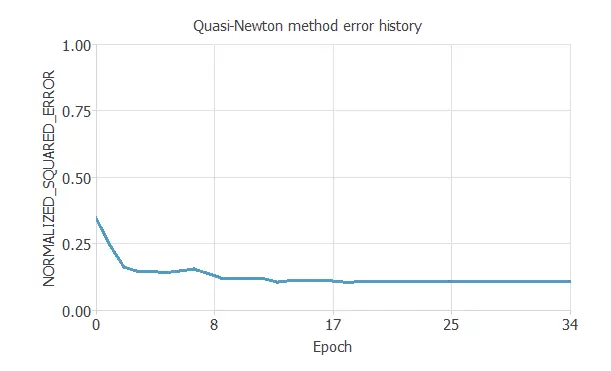# E-Nose to classify alcohol using Neural Designer

The aim is to classify five types of alcohols (1-Octanol, 1-Propanol, 2-Butanol, 2-Propanol, 1-Isobutanol) from the QCM sensor data.

A QCM is an electromechanical oscillator that contains a thin slice of quartz crystal with two channels placed on its surface. This physical device is sensitive to the resonance frequency.

The central goal here is to design an electronic nose model that makes proper classifications for the different alcohol types.

Electronic noses are sensors designed to mimic humans' sensory abilities to detect complex mixtures of chemical substances and biological origin.### Contents:

1. Application type.
2. Data set.
3. Neural network.
4. Training strategy.
5. Model selection.
6. Testing analysis.
7. Model deployment.

This example is solved with Neural Designer. To follow it step by step, you can use the free trial.

## 1. Application type

This is a classification project, since the variable to be predicted is categorical (1-Octanol, 1-Propanol, 2-Butanol, 2-Propanol, 1-Isobutanol).

## 2. Data set

The first step is to prepare the data set, which is the source of information for the classification problem. For that, we need to configure the following concepts:

• Data source.
• Variables.
• Instances.

The data source is the file QCMalcoholsensor.csv. It contains the data for this example in comma-separated values (CSV) format. The number of columns is 6, and the number of rows is 26.

• freq_1: Meseaured frecuency for concentration 1: Air ratio (ml): 0.799 Gas ratio(ml):0.201
• freq_2: Meseaured frecuency for concentration 2: Air ratio (ml): 0.700 Gas ratio(ml):0.300
• freq_3: Meseaured frecuency for concentration 3: Air ratio (ml): 0.600 Gas ratio(ml):0.400
• freq_4: Meseaured frecuency for concentration 4: Air ratio (ml): 0.501 Gas ratio(ml):0.499
• freq_5: Meseaured frecuency for concentration 5: Air ratio (ml): 0.400 Gas ratio(ml):0.600
• class: 1-Octanol, 1-Propanol, 2-Butanol, 2-Propanol, 1-Isobutanol used as the target.

The input variables are the five first variables. They are the five different concentrations through which each of the different alcohols is passed through the sensor.

Note that neural networks work with numbers. In this regard, the categorical variable "class" is transformed into three numerical variables as follows:

• 1-Octanol: 1 0 0 0 0.
• 1-Propanol: 0 1 0 0 0.
• 2-Butanol: 0 0 1 0 0.
• 2-Propanol: 0 0 0 1 0.
• 1-Isobutanol: 0 0 0 0 1.

The instances are divided into training, selection, and testing subsets. They represent 60% (15), 0% (0), and 20% (25) of the original instances, respectively, and are split at random.

We can calculate the distributions of all variables. The next figure is the pie chart for the alcohols types.As we can see, the target is well-distributed since there is the same number for the five different alcohols types.

Finally, the inputs-targets correlations might indicate to us what factors most influence.As we can see, the variables are strongly correlated.

## 3. Neural network

The second step is to choose a neural network. For classification problems, it is usually composed by:

• A scaling layer.
• Two perceptron layers.
• A probabilistic layer.

The scaling layer contains the statistics on the inputs calculated from the data file and the method for scaling the input variables. Here the minimum and maximum method has been set. Nevertheless, the mean and standard deviation method would produce very similar results.

In our case there is no a perceptron layer. This is due to having little data, so we simplify the program.

The probabilistic layer allows the outputs to be interpreted as probabilities. In this regard, all outputs are between 0 and 1, and their sum is 1. The softmax probabilistic method is used here.

The neural network has five outputs since the target variable contains 5 classes (1-Octanol, 1-Propanol, 2-Butanol, 2-Propanol, 1-Isobutanol).

## 4. Training strategy

The fourth step is to set the training strategy, which is composed of:

• Loss index.
• Optimization algorithm.

The loss index chosen for this application is the normalized squared error with L2 regularization.

The error term fits the neural network to the training instances of the data set. The regularization term makes the model more stable and improves generalization.

The optimization algorithm searches for the neural network parameters which minimize the loss index. The quasi-Newton method is chosen here.

The following chart shows how the training and selection errors decrease with the epochs during the training process.The final value are training error = 0.109 NSE, and the selection error doesn't appear because the instances are divided into training and testing subsets.

## 5. Model selection

The objective of model selection is to find the network architecture with the best generalization properties, that is, that which minimizes the error on the selected instances of the data set.

Order selection algorithms train several network architectures with a different number of neurons and select that with the smallest selection error.

The incremental order method starts with a small number of neurons and increases the complexity at each iteration.

## 6. Testing analysis

The purpose of the testing analysis is to validate the generalization performance of the model. Here we compare the neural network outputs to the corresponding targets in the testing instances of the data set.

In the confusion matrix, the rows represent the targets (or real values) and the columns the corresponding outputs (or predictive values). The diagonal cells show the correctly classified cases, and the off-diagonal cells show the misclassified cases.

Predicted 1-Octanol Predicted 1-Propanol Predicted 2-Butanol Predicted 2-Propanol Predicted 1-Isobutanol
Real 1-Octanol 1 (10.0%) 0 0 0 0
Real 1-Propanol 0 3 (30.0%) 0 0 0
Real 2-Butanol 0 0 3 (30.0%) 0 0
Real 2-Propanol 0 0 0 1 (10.0%) 0
Real 1-Isobutanol 0 0 0 0 2 (20.0%)

As we can see, the number of instances that the model can correctly predict is 10 (100%), so there are no misclassified cases. This shows that our predictive model has an excellent classification accuracy.

## 7. Model deployment

The neural network is now ready to predict outputs for inputs that it has never seen. This process is called model deployment.

To classify a given alcohol, we calculate the neural network outputs from the frequencies corresponding to the different types of concentration. For instance:

• Freq_1: -54.764 Hz.
• Freq_2: -90.826 Hz.
• Freq_3: -132.372 Hz.
• Freq_4: -173.337 Hz.
• Freq_5: -220.833 Hz.
• Probability of 1-Octanol: 1.5 %.
• Probability of 1-Propanol: 9.6 %.
• Probability of 2-Butanol: 1.3 %.
• Probability of 2-Propanol: 24.2 %.
• Probability of 1-Isobutanol: 63.4 %.

For this particular case, our e-nose developed would classify the alcohol as 1-Isobutanol, since it has the highest probability.

The mathematical expression of the trained neural network is listed below.

scaled_freq_1 = freq_1*(1+1)/(1.175490007e-38-(-86.33999634))+86.33999634*(1+1)/(1.175490007e-38+86.33999634)-1;
scaled_freq_2 = freq_2*(1+1)/(1.175490007e-38-(-129.7100067))+129.7100067*(1+1)/(1.175490007e-38+129.7100067)-1;
scaled_freq_3 = freq_3*(1+1)/(1.175490007e-38-(-183.9400024))+183.9400024*(1+1)/(1.175490007e-38+183.9400024)-1;
scaled_freq_4 = freq_4*(1+1)/(1.175490007e-38-(-231.0800018))+231.0800018*(1+1)/(1.175490007e-38+231.0800018)-1;
scaled_freq_5 = freq_5*(1+1)/(1.175490007e-38-(-296.6799927))+296.6799927*(1+1)/(1.175490007e-38+296.6799927)-1;

probabilistic_layer_combinations_0 = 3.41873 +2.6168*scaled_freq_1 +2.38179*scaled_freq_2 +2.38347*scaled_freq_3 +2.32046*scaled_freq_4 +2.43974*scaled_freq_5
probabilistic_layer_combinations_1 = -2.23754 +3.38886*scaled_freq_1 -0.654598*scaled_freq_2 +1.7727*scaled_freq_3 -4.21105*scaled_freq_4 -3.60481*scaled_freq_5
probabilistic_layer_combinations_2 = -7.19512 -0.314361*scaled_freq_1 -1.66506*scaled_freq_2 -6.35273*scaled_freq_3 -1.9822*scaled_freq_4 -1.74316*scaled_freq_5
probabilistic_layer_combinations_3 = 1.93658 -2.57851*scaled_freq_1 -4.05779*scaled_freq_2 -2.48873*scaled_freq_3 +2.02679*scaled_freq_4 +6.56201*scaled_freq_5
probabilistic_layer_combinations_4 = 4.05001 -3.09451*scaled_freq_1 +3.95852*scaled_freq_2 +4.65626*scaled_freq_3 +1.84598*scaled_freq_4 -3.60918*scaled_freq_5

sum_ = exp(probabilistic_layer_combinations_0 + exp(probabilistic_layer_combinations_1 + exp(probabilistic_layer_combinations_2 + exp(probabilistic_layer_combinations_3 + exp(probabilistic_layer_combinations_4;

1-Octanol = exp(probabilistic_layer_combinations_0)/sum_;
1-Propanol = exp(probabilistic_layer_combinations_1)/sum_;
2-Butanol = exp(probabilistic_layer_combinations_2)/sum_;
2-Propanol = exp(probabilistic_layer_combinations_3)/sum_;
1-Isobutanol = exp(probabilistic_layer_combinations_4)/sum_;


We have developed an e-nose algorithm that can be implemented in sensors, like QCM sensors, to detect the corresponding type of alcohol.

## References:

• UCI Machine Learning Repository. QCM12 Data Set.
• M. Fatih Adak, Peter Lieberzeit, Purim Jarujamrus, Nejat Yumusak, Classification of alcohols obtained by QCM sensors with different characteristics using ABC based neural network, Engineering Science and Technology, an International Journal, 2019, ISSN 2215-0986. Web Link.